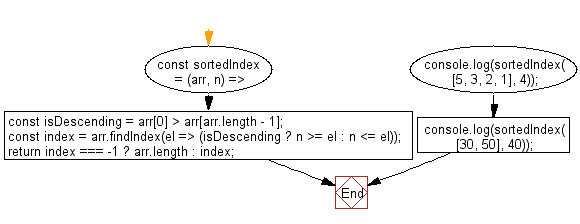# JavaScript Sorting Algorithm: Sorted Index

## JavaScript Sorting Algorithm: Exercise-22 with Solution

Write a JavaScript program to find the lowest index at which a value should be inserted into an array to maintain its sorting order.

• Loosely check if the array is sorted in descending order.
• Use Array.prototype.findIndex() to find the appropriate index where the element should be inserted.

Sample Solution:

JavaScript Code:

``````const sortedIndex = (arr, n) => {
const isDescending = arr > arr[arr.length - 1];
const index = arr.findIndex(el => (isDescending ? n >= el : n <= el));
return index === -1 ? arr.length : index;
};
console.log(sortedIndex([5, 3, 2, 1], 4));
console.log(sortedIndex([30, 50], 40));
```
```

Sample Output:

```1
1
```

Flowchart:Live Demo:

See the Pen javascript-common-editor by w3resource (@w3resource) on CodePen.

Improve this sample solution and post your code through Disqus

What is the difficulty level of this exercise?

Test your Programming skills with w3resource's quiz.

﻿